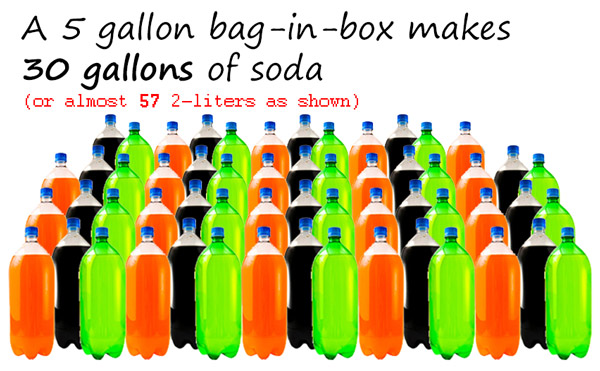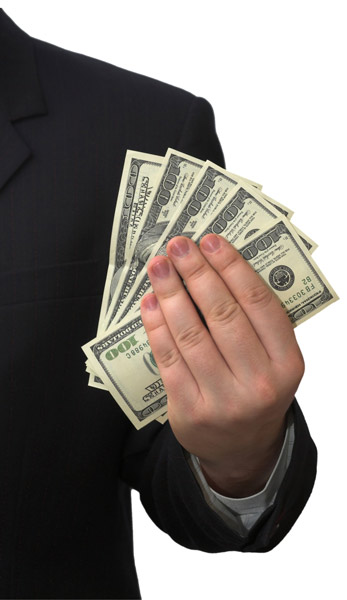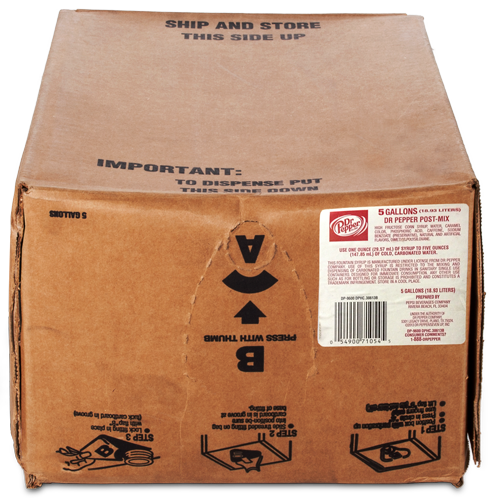# Why Own A Soda Fountain System?

## A: It can pay for itself in 1 month!• 30 gallons makes 384 12 oz glasses with ice
• Sell each glass for \$1.50 and you'll make \$576
• Each box is about \$60, so your profit is \$516 each box!
• Sell 32 glasses per day for a month (2.5 boxes) for a profit of \$1,548!

## 5-Gallon Bag-in-Box Guide

"How many drinks will I get from one five-gallon box of syrup?"

Although there is not a fixed number, here's an easy way to estimate how many drinks you would get from one 5-gallon BIB.1. Your soda bag holds 5 gallons of syrup.
2. There are 128 ounces in 1 gallon. So, there are 640 ounces in 5 gallons.
3. Your soda fountain system will mix water and syrup at a 5 to 1 ratio.
4. Divide cup size by 6 for number of ounces of syrup used per cup.
Example for 10 oz. cup: 10 ÷ 6 = approximately 1.7
There are approximately 1.7 oz. of syrup used per each 10 oz. cup
5. Divide ounces. of syrup used per cup by 640.
Example: 640 ÷ 1.7 = approximately 376
6. This is the estimated number of servings per 5 gallon bag in box, not factoring in ice**

**These estimates do not account for the amount of ice in each cup. More ice per cup would require less syrup, thus more servings per box of syrup.

### Estimated Servings Based on Cup Size

Cup Size Total oz. of syrup used Total number of servings per 5-gallon BIB**
7 oz. 1 to 2 640
9 oz. 1 to 2 320 to 640
10 oz. 2 320
12 oz. 2 to 3 214 to 320
16 oz. 3 214
20 oz. 4 160
24 oz. 4 to 5 128 to 160
32 oz. 6 to 7 107 to 128